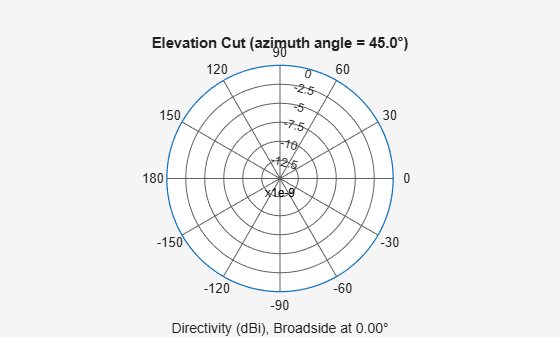Documentation

# directivity

System object: phased.IsotropicHydrophone
Package: phased

Directivity of isotropic hydrophone

## Syntax

```D = directivity(hydrophone,FREQ,ANGLE) ```

## Description

`D = directivity(hydrophone,FREQ,ANGLE)` returns the Directivity of the isotropic hydrophone, `hydrophone`, at frequencies specified by `FREQ` and in direction angles specified by `ANGLE`.

## Input Arguments

expand all

Isotropic hydrophone, specified as a `phased.IsotropicHydrophone` System object.

Example: `phased.IsotropicHydrophone`

Frequencies for computing directivity and patterns, specified as a positive scalar or 1-by-L real-valued row vector. Frequency units are in hertz.

• For an antenna, microphone, or sonar hydrophone or projector element, `FREQ` must lie within the range of values specified by the `FrequencyRange` or `FrequencyVector` property of the element. Otherwise, the element produces no response and the directivity is returned as `–Inf`. Most elements use the `FrequencyRange` property except for `phased.CustomAntennaElement` and `phased.CustomMicrophoneElement`, which use the `FrequencyVector` property.

• For an array of elements, `FREQ` must lie within the frequency range of the elements that make up the array. Otherwise, the array produces no response and the directivity is returned as `–Inf`.

Example: `[1e8 2e6]`

Data Types: `double`

Angles for computing directivity, specified as a 1-by-M real-valued row vector or a 2-by-M real-valued matrix, where M is the number of angular directions. Angle units are in degrees. If `ANGLE` is a 2-by-M matrix, then each column specifies a direction in azimuth and elevation, `[az;el]`. The azimuth angle must lie between –180° and 180°. The elevation angle must lie between –90° and 90°.

If `ANGLE` is a 1-by-M vector, then each entry represents an azimuth angle, with the elevation angle assumed to be zero.

The azimuth angle is the angle between the x-axis and the projection of the direction vector onto the xy plane. This angle is positive when measured from the x-axis toward the y-axis. The elevation angle is the angle between the direction vector and xy plane. This angle is positive when measured towards the z-axis. See Azimuth and Elevation Angles.

Example: `[45 60; 0 10]`

Data Types: `double`

## Output Arguments

expand all

Directivity, returned as an M-by-L matrix. Each row corresponds to one of the M angles specified by `ANGLE`. Each column corresponds to one of the L frequency values specified in `FREQ`. Directivity units are in dBi where dBi is defined as the gain of an element relative to an isotropic radiator.

## Examples

expand all

Compute the directivity of an isotropic hydrophone in different directions. Assume the signal frequency is 3 kHz. First, set up the hydrophone parameters.

```fc = 3e3; hydrophone = phased.IsotropicHydrophone('FrequencyRange',[1,10]*1e3, ... 'VoltageSensitivity',[-100,-90,-100]); patternElevation(hydrophone,fc,45)```First, select the angles of interest to be constant elevation angle at zero degrees. The five azimuth angles are centered around boresight (zero degrees azimuth and zero degrees elevation).

`ang = [-20,-10,0,10,20; 0,0,0,0,0];`

Compute the directivity along the constant elevation cut.

`d = directivity(hydrophone,fc,ang)`
```d = 5×1 0 0 0 0 0 ```

The directivity of an isotropic hydrophone is zero in every direction.